## 什么是全导数？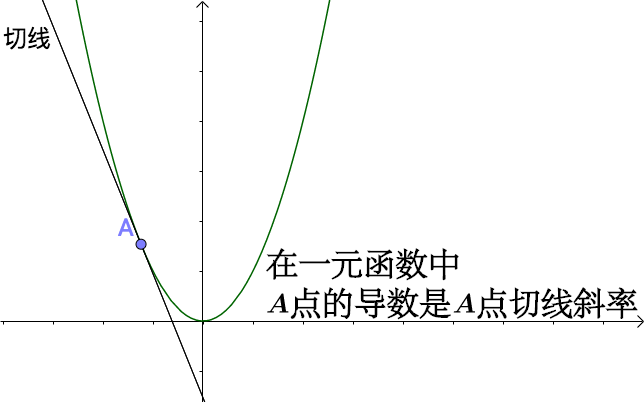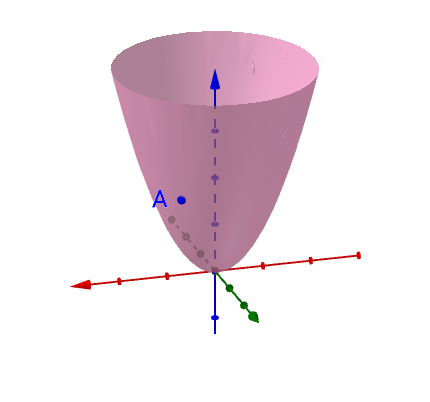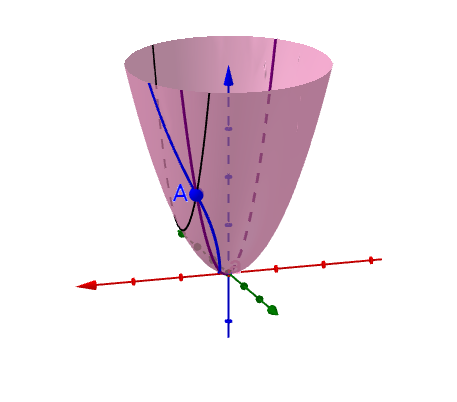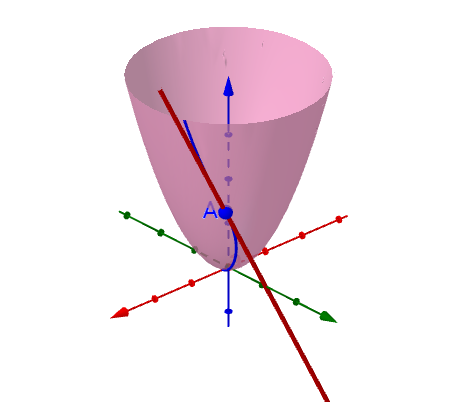• 方向导数、偏导数是特殊的全导数
• 每一根切线都和一个全导数“相关”，这个“相关”是什么意思？难道不就是切线的斜率就是全导数吗？

1 参数方程

1.1 通过参数方程来描述所有的曲线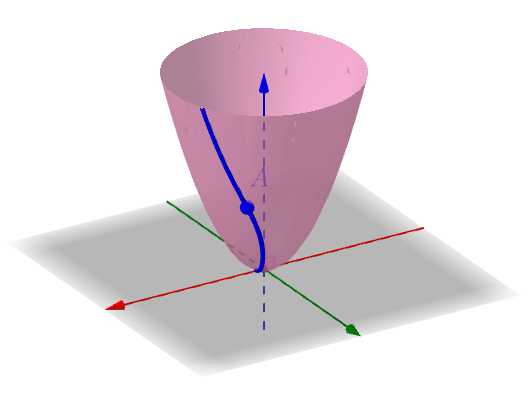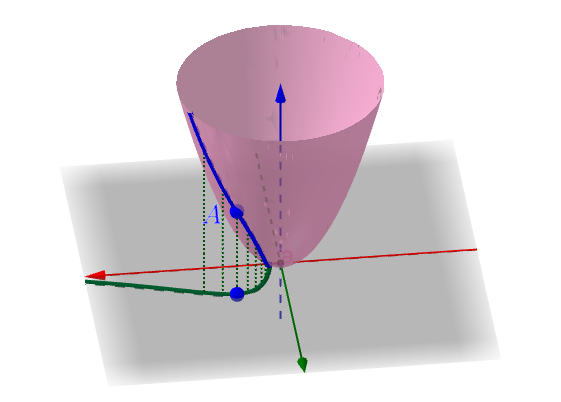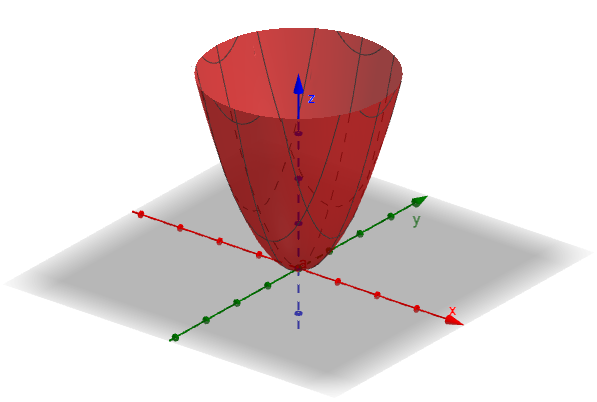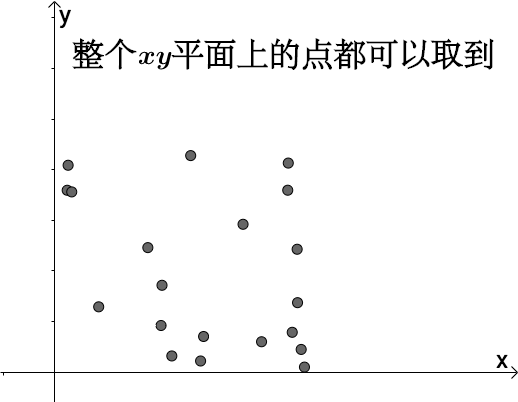$$\begin{cases} z=x^2+y^2\\ x=t\\ y=t\end{cases}$$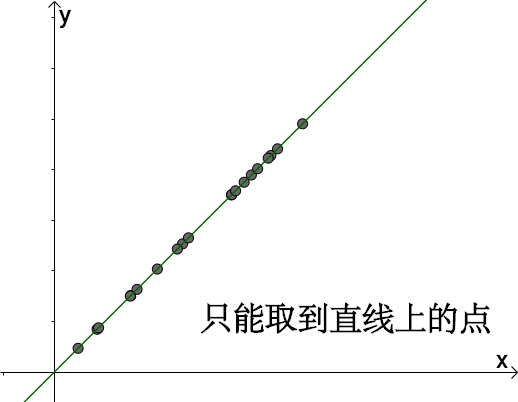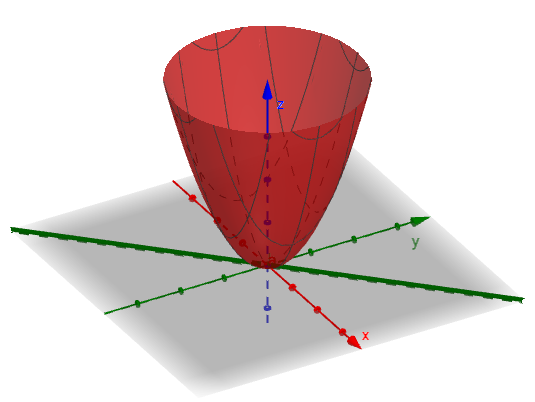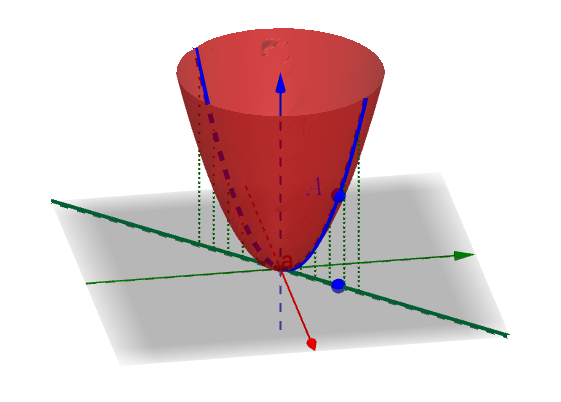$$\begin{cases} z=x^2+y^2\\ x=t\\ y=t\end{cases}$$

1.2 参数方程可以拍扁三维图像$$\begin{cases} z=x^2+y^2\\ x=t\\ y=t\end{cases}$$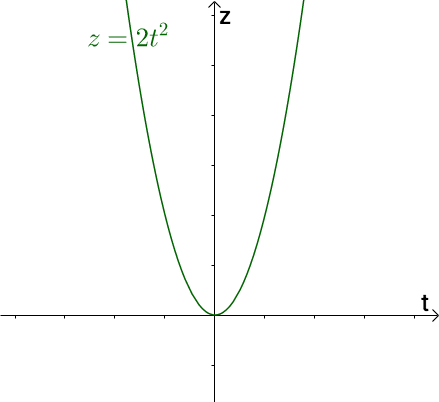2 全导数、偏导数、方向导数

2.1 全导数

$xy$平面上有很多不同的曲线，这些曲线也总能决定曲面上的曲线。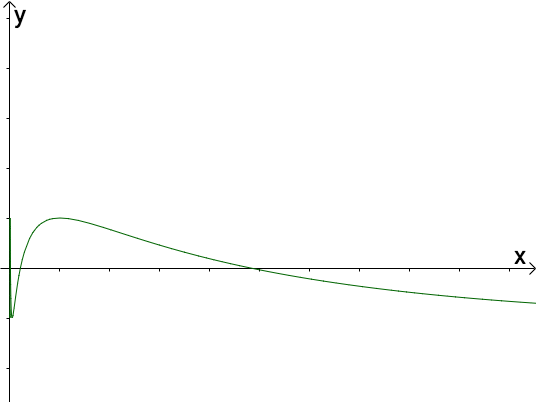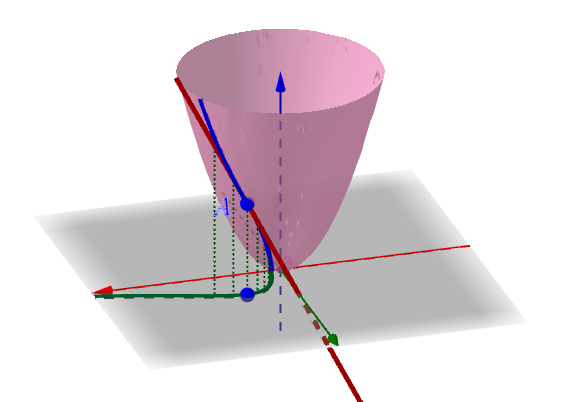2.2 偏导数

$xy$平面上不同类型的曲线中，有一种平行于$x$轴或者$y$轴的直线（以平行于$x$轴举例）：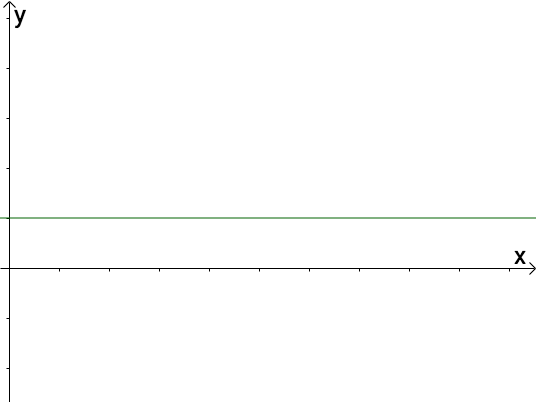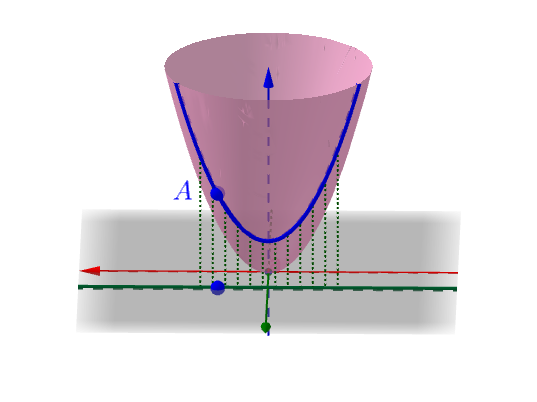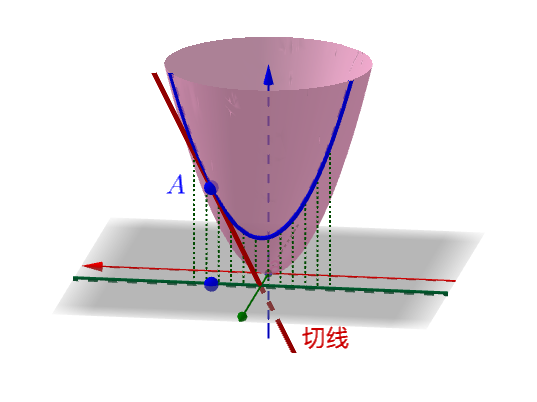2.3 方向导数

$xy$平面不光有平行于坐标轴的直线，还有各种射线，由这些射线决定的曲线：